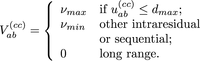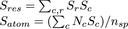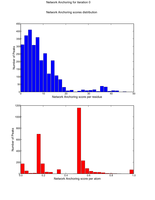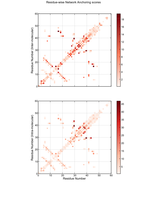##### Personal tools
You are here: Home / Network anchoring

# Network anchoring

Warning: This example is for ARIA >= 2.2 only.

## Background

The network-anchoring approach was proposed by ((Herrmann et al. 2002) in order to reduce the number of possibilities of NOE peak assignment during the iterative NMR structure refinement with CANDID and CYANA (G  ̈untert, 2004). The approach is based on the ranking of each NOE assignment, using the information about the assignments of neighbourig nuclei in the 3D space. It is based on the hypothesis that the correctly assigned restraints form a self- consistent subset of the network of restraints. The network-anchoring approach is new in ARIA, as, in previous versions, it was not present, nor in the python code, neither in the CNS Fortran code. It was implemented in the following way, which is an adaptation of (Herrmann et al., 2002) to the ARIA philosophy. The network anchoring is indeed used to calculate a contribution score, which replaces the usual contribution weight of the ARIA protocol.

A preliminary analysis over all amino-acids and possible sequences of two amino-acids determined the list of spins pairs separated by at most two dihedral angles, and whose the distance is smaller than 5.5  A, whatever are the dihedral angle values. The analysis was not performed using a geometric formal calculation as previously done in the literature (W  ̈uthrich et al., 1983), but by an exhaustive sampling of possible conformations of the amino-acids and sequences of two amino-acids, using the CNS topology definition and only allowing dihedral angle rotations. All the possible ”covalent” distances are stored in a XML file.

At the beginning of the ARIA calculation, the network is created: for each pair of spins α, β, it contains all protons γ of the residue range [r − 1,r + 1],
conneted to either α or β with an initial assignments, and where r is the residue number of the spin α or β. For a given pair of spins a, b belonging to the α, β network, the covalent score describes the covalent structure between these spins. If a,b belongs to the list of spins pairs previously determined by the exhaustive sampling of possible amino-acid conformations, the covalent score  Vab(cc) is:The parameters Vmax and Vmin values are built-in and set to values 1.0 and 0.1, respectively.

The number of indirect connections between the spins α and β via the atoms γ belonging to the α, β network is calculated as:(4)

where vαγ = max(V αγ(cc) , V αγvol ) if max(Vαγ(cc) , V αγvol ) > Vmin or 0.0 otherwise.

V αγvol  is the volume of the corresponding NOE assignments between the spins α and γ.

The network score Nc for each contribution c is calculated as the mean  of the values Nαβ obtained (Eq. 4) for each spin pair α, β included into the contribution. Finally, the contribution scores Nc are normalized.

From the network contribution score Nc , the contribution score Sc , which will be used then as the contribution weight, is obtained from the product of the value Nnormc  and the weight wc  determined the distance observed in the structures calculated in the previous iteration. This product is also normalized:

The contribution weigths are updated with the new score values Sc . Scores Sres and Sato depending on the residues and atoms are then derived from the contributions scores Nc , in order to apply criteria allowing to keep or to reject an AriaPeak. First, the scores Nc are summed over each residue pairs involved in this a peak to obtain residue score Sr . For each AriaPeak, a residue score Sres and an atom score Sato are calculated:where r are the residue pairs and c  the contributions involved in the peak, and nsp is the number of spin pairs.

Using the parameters Sres and Satom , the peak is declared active and therefore conserved if one of the two following rules is verified:

• SresNhigh res
• SresNmin res   &&   SatomNmin ato

Thus, the network-anchoring is used to

• Remove possible noise peaks which have low Sres and Satom scores.
• Reduce the ambiguity within each peak by eliminating contributions with low weight Sc

The two rules previously described are corresponding to the rules proposed in the original publication about network-achoring (Herrmann et al, 2002). In CANDID (Herrman et al, JMB 2002), a peak p is kept if one of the two following conditions (page 215, Elimination of spurious NOE cross-peaks'') is verified:

• < \overline{N} >p  >=  \overline{N}high
• < \overline{N} >p  >=  \overline{Nmin}  &&  <N>p  >= Nmin
where \overline{N} stands for a overlined N.

The value of \overline{N}high is constant and equal to 4.0 (Table 4, page 225, Herrman et al, JMB 2002) and the values of \overline{Nmin}
and Nmin vary (Table 5,  page 225, Herrman et al, JMB 2002). For a run with 7 iterations, \overline{Nmin} is equal to 1.0 at the first iteration, equal to 0.75 at the second iteration and equal to 0.5 in the following iterations. In the same run, Nmin is equal to 0.25 in the two first iterations, and is then set to 0.4.

The parameters Nhigh res , Nmin res and Nmin ato of ARIA correspond thus to the parameters \overline{Nhigh}, \overline{Nmin} and Nmin in CANDID.

## Example description

One example of the use of the network-anchoring procedure is given for the Tudor domain (ref):

## Network-anchoring parameters

In the project xml file, in each section <iteration> ... </iteration>, the following line:

<network_anchoring 	high_residue_threshold="4.0" enabled="no" min_residue_threshold="1.0" min_atom_threshold="0.25"/>

contains the network-anchoring parameters. Here are the definitions:

• enabled specifies if the network-anchoring must be used during a particular iteration.
• high_residue_threshold : Minimal residue-wise network-anchoring score for a peak to be active (Nhigh res )

• min_residue_threshold Minimal network-anchoring score per residue for a peak to be active. (Nmin res)

• min_atom_threshold Minimal network-anchoring score per atoms for a peak to be active. (Nmin ato )

Network-anchoring is especially usefull during the first iterations of the ARIA calculation (i.e it 0 to 3). Netwok-anchoring parameters can be changed for each iteration in order to decrease the stringence and avoid wrong assigments.

## Network-anchoring results

At the end of the network-anchoring analysis of each iteration, ARIA saves the result in different files:
• noe_restraints.network : a text file which contains the Nres and Nato scores of each peak.
• 2 Postcript files: graphics/network_dist.ps and graphics/network_2D.ps
• network_dist.ps reports the distribution of the Nres (blue) and Nato (red) scores
• network_2D.ps presents the sum of Nres scores for pairs of residues on a contact map with a color sclae (left). If the molecule contains two chains, the intermolcular and intramolecular contacts are separated on two maps. (see figures)

network-anchoring
scores distribution
network_dist.ps
Network--anchoring
scores map
network_2D.ps« May 2021 »
May
MonTueWedThuFriSatSun
12
3456789
10111213141516
17181920212223
24252627282930
31